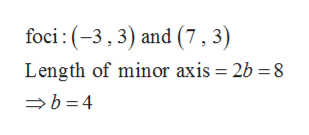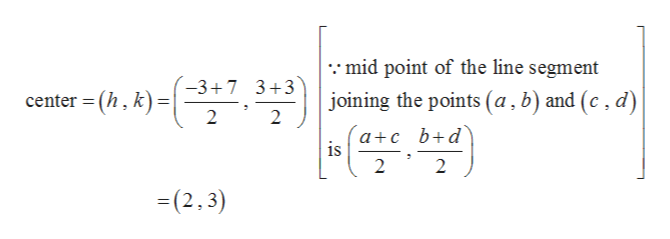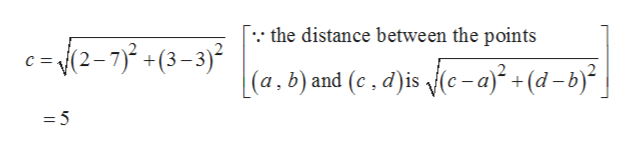# For Exercise, write the standard form of an equation of an ellipse subject to the given conditions.  Foci: (−3, 3) and (7, 3); Length of minor axis: 8 units

Question
1 views

For Exercise, write the standard form of an equation of an ellipse subject to the given conditions.

Foci: (−3, 3) and (7, 3); Length of minor axis: 8 units

check_circle

Step 1

Given,help_outlineImage Transcriptionclosefoci :(-3, 3) and (7 , 3) Length of minor axis = 2b =8 → b = 4 fullscreen
Step 2

Now centre of the ellipse is the mid point of the foci, that ishelp_outlineImage Transcriptionclose::mid point of the line segment -3+7 3+3 (2,) joining the points (a, b) and (c , d) center = (h, k) = a+c _b+d° is 2 2 =(2,3) fullscreen
Step 3

Now the distance between the cent...help_outlineImage Transcriptionclose[: the distance between the points c= {(2-7)? +(3–3)² [(a,b) and (c , d)is (c - a)² + (d – b)² = 5 fullscreen

### Want to see the full answer?

See Solution

#### Want to see this answer and more?

Solutions are written by subject experts who are available 24/7. Questions are typically answered within 1 hour.*

See Solution
*Response times may vary by subject and question.
Tagged in

### Other